学哥量化交易学习 持续学习，提高认知，磨炼技艺，从零基础学编程并跨过量化程序交易的大门。 返回首页

0010 人生就像死循环，只要不退出就要继续运行

```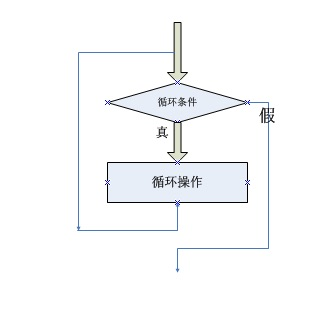while循环语法

while 条件表达式为True:
循环操作1
循环操作2

name=input()
while name<>"michael":
name=input()
print name

#coding=utf-8
#从1加到100求和
#作者：学哥  时间：2017/1/1

#变量c作为计数器
c=1
#变量total保存累加值
total=0
#循环100次，如果计数器大于100则退出循环
while c<=100:
#计数器同时作为累加增加的值
total = total + c
#将计数器加1
c = c + 1
print "total 1-100:",totalc=1
total=0

total=0+1=1
c=1+1=2

total=1+2=3
c=2+1=3

total=3+3=6
c=3+1=4

total=6+4=10
c=4+1=5

total=10+5=15
c=5+1=6
......

total=4851+99=4950
c=99+1=100

total=4950+100=5050
c=100+1=101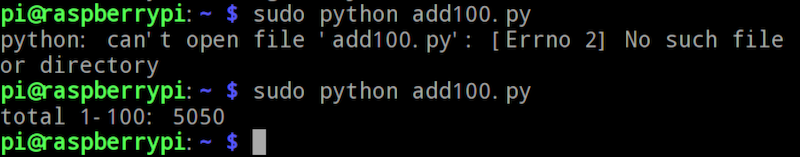#coding=utf-8
#从1开始连续100个奇数相加求和
#作者：学哥  时间：2017/1/1

#变量c作为计数器
c=1
#变量jishu保存奇数
jishu=1
#变量total保存累加值
total=0
#循环100次，如果计数器大于100则退出循环
while c<=100:
#奇数作为累加增加的值
total = total + jishu
#奇数增加2，变成下一个奇数
jishu = jishu + 2
#将计数器加1
c = c + 1
print "total 100 jishu:",total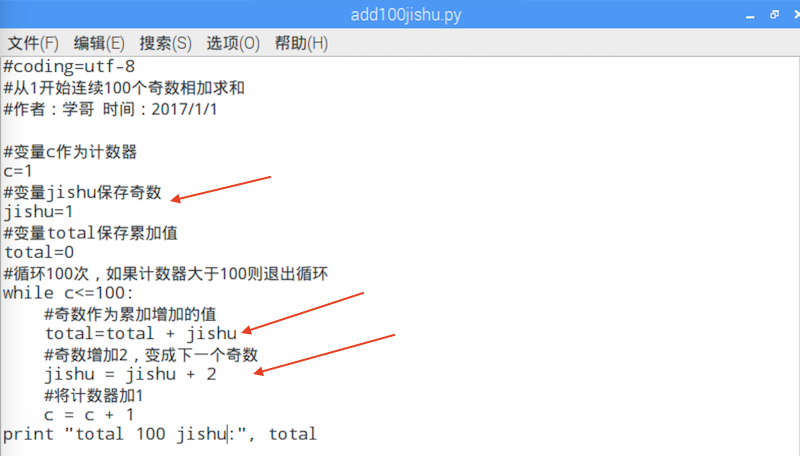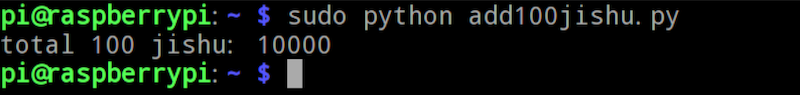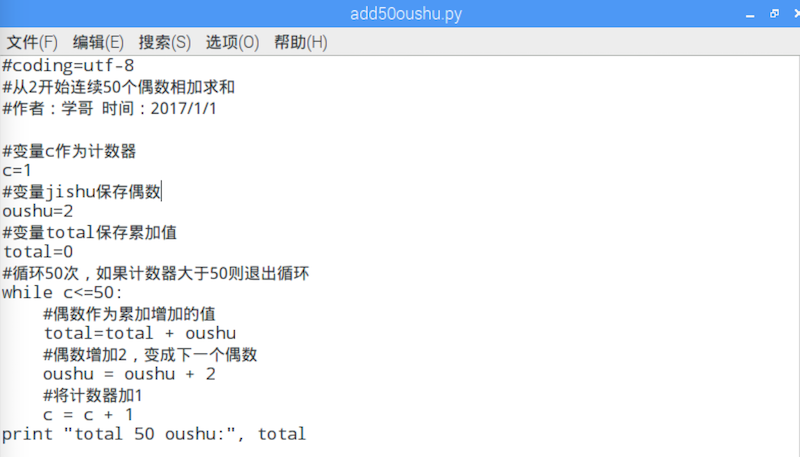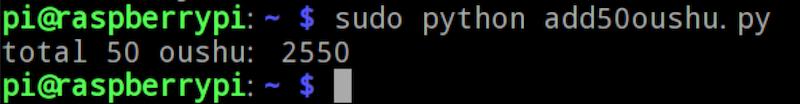while 条件表达式为True:
循环操作1
break
循环操作2

while 条件表达式为True:
循环操作1
if 条件表达式2为True:
break
循环操作2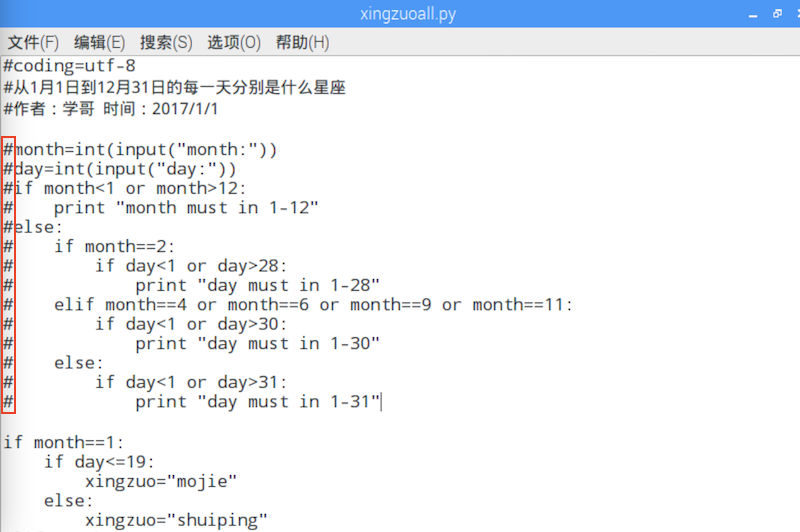month = 1
day = 1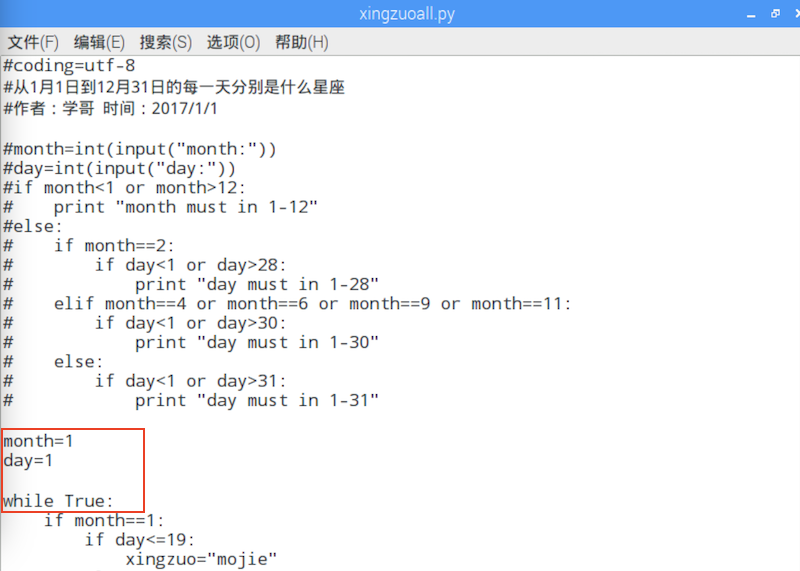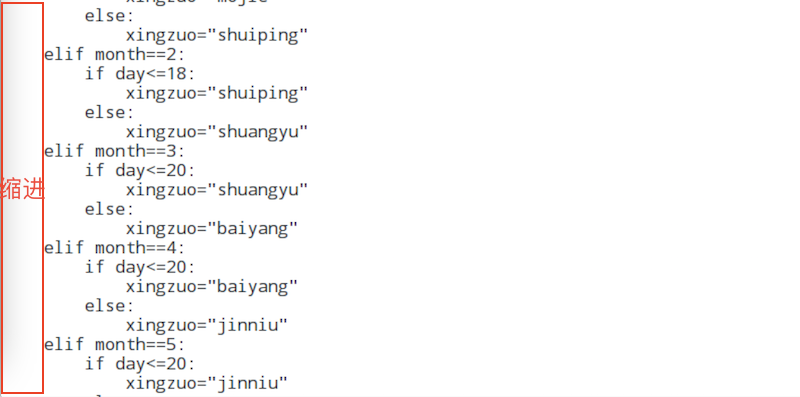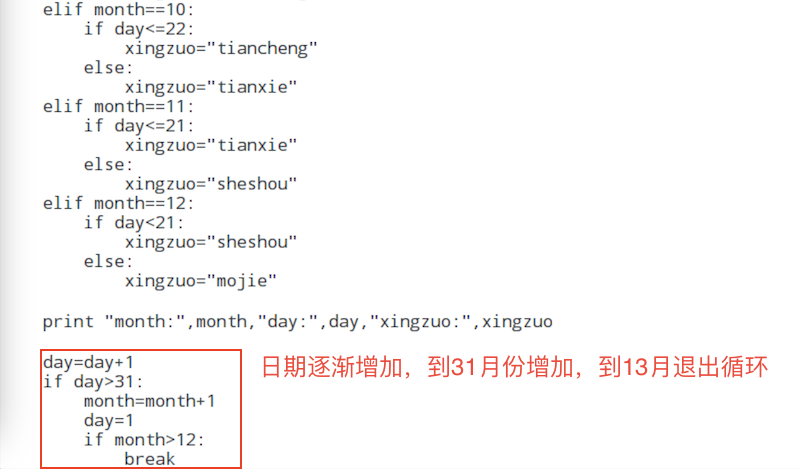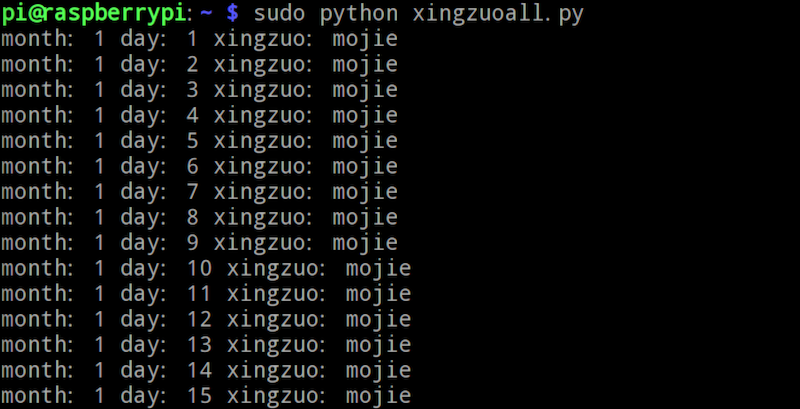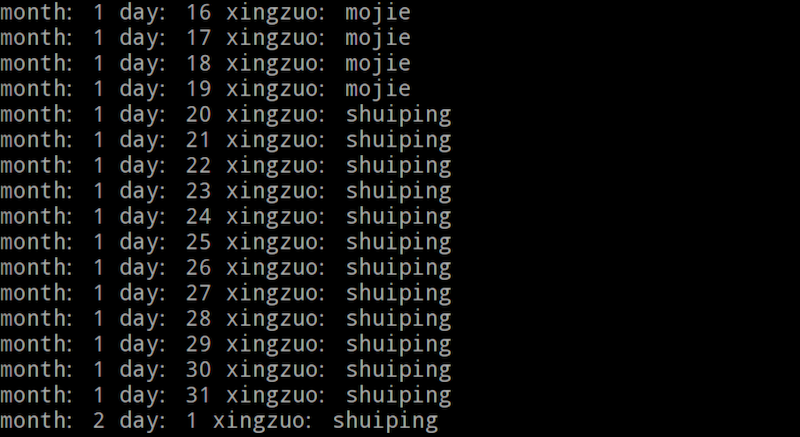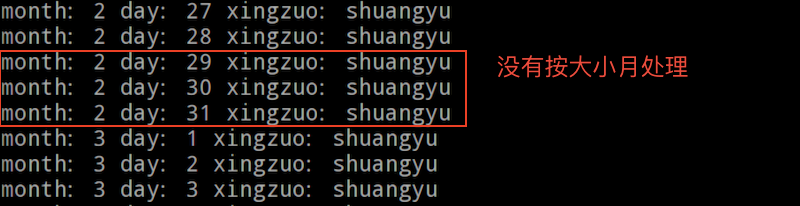maxday初始值给31，然后根据当前month修改maxday为28或者30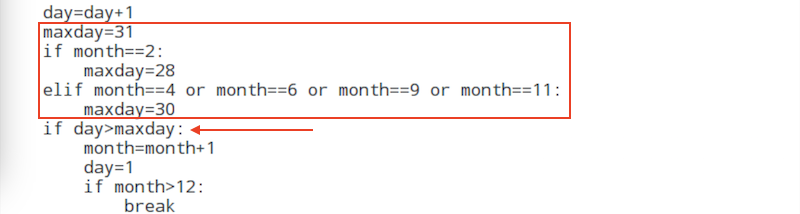```学哥量化交易学习团队 致力于：持续学习，提高认知，磨炼技艺，持续输出高质量的教程和课程，帮助更多的人从零基础学编程并跨过量化程序交易的大门。 零基础学编程Python入门课程 课程包含以下内容： 1.图文教程《零基础学编程入门-从Python到Web网站.pdf》，学哥原创版权，共计1024页高清图文教程。 2.视频课程《零基础学编程Python入门》，学哥原创版权，共计24节课45个高清视频。 3.视频教程《玩转魔方》，学哥原创版权，可以送给孩子，共计16个高清视频。 4.图文教程《如何寻找编程相关的电子书下载资源.pdf》。 5.加入学哥编程学习辅导QQ群在线交流。 客服 10:00-17:00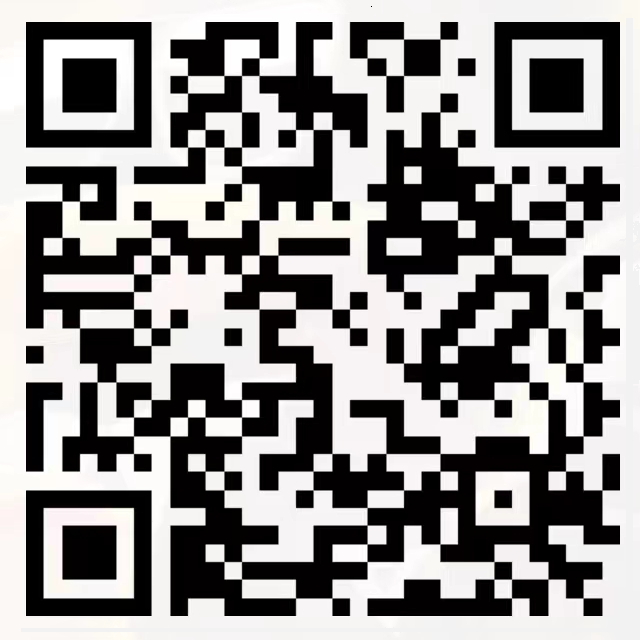QQ: 44556287 微信公众号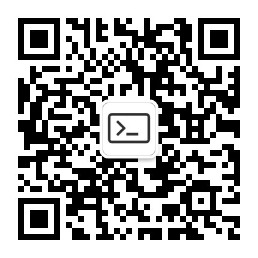学哥量化交易学习

 微信公众号：学哥量化交易学习     QQ群1：603559164    客服QQ号：44556287 工信部备案/许可证编号：沪ICP备15050545号-4